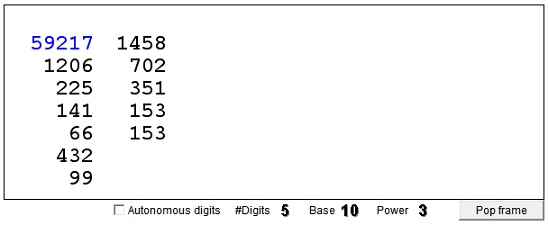# Sums of Powers of Digits

Given numbers N and m, compute the sum of digits of N raised to the power of m. For example, let N = 1127 and m = 2. Compute the new number from 12 + 12 + 22 + 72 = 1 + 1 + 4 + 49 = 55. Apply the same procedure to the new number: 52 + 52 = 25 + 25 = 50. Continue in this manner. The numbers are said to be obtained iteratively from one another, and their sequence is said to be an iterative process. A question of interest is what happens when you run an iterative process long enough.

In the decimal system, squaring of digits may only result in cycles. I.e., from a certain point on the process starts repeating itself. Raising digits to the third power and adding up leads to one of 5 points: 1, 153, 370, 371, 407. Modulo 3 those numbers are 1, 0, 1, 2, 2. All whole numbers divisible by 3 eventually settle on 153. Function f in this case has the property that it preserves the value modulo 3. For example, all iterates of a number equal 2 modulo 3 are equal 2 modulo 3. Far as I can judge, such numbers settle on either 371 or 407. On the other hand, there are cycles of numbers equal to 1 (mod 3). For example 23 + 43 + 43 = 136, while 13 + 33 + 63 = 244. Also f(160) = 217, f(217) = 352, and f(352) = 160, but there are more of them. Iterations that converge starting with numbers equal 1 (mod 3) settle on either 1 or 370.

### If you are reading this, your browser is not set to run Java applets. Try IE11 or Safari and declare the site https://www.cut-the-knot.org as trusted in the Java setup.What if applet does not run?

A few more words